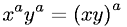Equations > Algebra > Exponents/Powers > Distributive Property of Exponents

### Distributive Property of ExponentsLatex Code:

MathML Code:

 ${x}^{a}{y}^{a}={\left(\mathrm{xy}\right)}^{a}$

MathType 5.0: